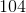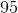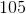# Computer Science : Common Data Structures

## Example Questions

### Example Question #1 : Lists

Which of the following is NOT a difference between the Array class and the ArrayList class in Java?

Arrays have a fixed size at creation, while ArrayLists can grow as needed.

All of the other answer choices are differences between Arrays and ArrayLists in Java.

You have to manually add things to an Array by calling a command similar to my_array[some_number] = some_value. With ArrayLists, you use the add() method.

Arrays can store both primitives and Objects, while ArrayLists can only store Objects.

To find the number of spots in an Array, you append .length to the end of it. To find the number of spots in an ArrayList, you use the size() method.

All of the other answer choices are differences between Arrays and ArrayLists in Java.

Explanation:

In Java, Array is a fixed length data structure, while ArrayList is a variable length Collection Class (other Collection class members include HashMap and HashSet). This means that an Array cannot change its size once it's made; a whole new Array must be made. ArrayLists can change size at will.

Arrays can reference both primitives (like int) and types (like Integer). ArrayLists can only reference types, not primitives. Through a method called "Autoboxing", it can appear that an ArrayList stores a primitive, but what it really is doing is automatically masking the primitive with the type it's like so that it works with the ArrayList.

During the creation of an Array, the .length value is assigned, so whenever you append .length to the end of an array, you get the length (which will never change for the given Array). For ArrayLists, you use the .size() function, which returns the length of the ArrayList. Because the ArrayList size is variable, it is a function (which is why the parenthesis are there at the end).

Java provides the add() function for ArrayLists, which, as the name implies, adds the argument to the ArrayList. Arrays, on the other hand, do not have functions to add elements. To add something to an Array, you'd call something similar to this:

int[] my_array = new int;
my_array = 5;

### Example Question #91 : Standard Data Structures

Write a program that iterates through this data structure and prints the data (choose the best answer):

List<List<String>> listOflistOfStrings = new ArrayList<ArrayList<String>>();

for (int i = 0; i < listOflistOfStrings.size(); i++) {

List<String> listOfStrings = listOflistOfStrings.get(i);

for (int j = 0; j < listOflistOfStrings.size(); j++) {

System.out.println(listOfStrings.get(j));

}

}

for (List<String> l : listOflistOfStrings) {

List<String> listOfStrings = l;

for (String s : l) {

System.out.println(s);

}

}

for (int i = 0; i < listOflistOfStrings.size(); i++) {

List<String> listOfStrings = listOflistOfStrings.get(i);

for (int j = 0; j < listOfStrings.size(); j++) {

System.out.println(listOfStrings.get(j));

}

}

for (List<String> l : listOflistOfStrings) {

List<String> listOfStrings = l;

for (List<String> s : l) {

System.out.println(s);

}

}

for (List<String> l : listOflistOfStrings) {

List<String> listOfStrings = l;

for (String s : l) {

System.out.println(s);

}

}

Explanation:

The correct answer uses a ForEach loop. A ForEach loop is recommended for iterating through Lists because Lists contain iterators. ForEach loops use the iterator to iterate through the List. One of the answers used a regular For loop, while the answer was correct, it was not the best choice.

### Example Question #1 : Lists

Consider the code below:

ArrayList<String> myPhilosophers = new ArrayList<String>();

for(int i = 0; i < myPhilosophers.size(); i++) {

String s = myPhilosophers.get(i);

if(s.charAt(0) >= 'H' || s.charAt(3) < 'd') {

myPhilosophers.set(i, s.toUpperCase());

}

}

System.out.println(myPhilosophers);

What is the output for the code above?

[FREGE, HUSSERL, HEGEL, BILL, FREDERICK]

[Frege, HUSSERL, HEGEL, Bill, FREDERICK]

[Frege, HUSSERL, HEGEL, Bill, Frederick]

[FREGE, Husserl, Hegel, Bill, FREDERICK]

[Frege, Husserl, Hegel, Bill, FREDERICK]

[Frege, HUSSERL, HEGEL, Bill, Frederick]

Explanation:

Consider the logic that is in the loop.  The if statement will be reached either if you:

1. Have a first character that is H or later in the alphabet (in capital letters).
2. Have a fourth character that is less than d

The first case applies to "Husserl" and "Hegel."  However, the second does not apply to any—not even to "Frederick", for d is equal  to d, not less than it!

### Example Question #1 : Lists

Consider the code below:

ArrayList<String> names = new ArrayList<String>();

for(int i = 0; i < 12; i++) {

for(int i = 0; i < 12; i++) {

if(names.get(i).equals("Frege")) {

names.remove(i);

}

}

What is the bug in the code above?

The list will overrun its indices in the second loop.

There are no bugs.

The .get method is buggy and likely to crash.  It is better to use an Iterator.

The list will use up all of the memory on the machine.

There is an infinite loop in one of the two loops.

The list will overrun its indices in the second loop.

Explanation:

In the second loop, when you remove one of the items, you thus make the ArrayList one element shorter.  This means that if you attempt to go through to index 11, you will receive an error at some point, for the list will have "shrunk".  This is like an array out of bounds error, though it will be an IndexOutOfBoundsException exception.

### Example Question #1 : Lists

Consider the following code:

public static class Circle {

public Circle(double r) {

}

public double getArea() {

}

}

public static void main(String[] args) {

ArrayList<Circle> circles = new ArrayList<Circle>();

for(int i = 0; i < 10; i++) {

circles.add(new Circle(i + 4 * 2));

}

}

Which of the following represents code for iterating through the list circles in order to output the areas of the circles contained therein?

for(int i = 0; i < circles.size; i++) {

System.out.println(circles.get()[i].getArea());

}

for(int i = 0; i < circles.size(); i++) {

System.out.println(circles.get(i).getArea());

}

for(int i = 0; i < circles.size(); i++) {

System.out.println(circles[i].getArea());

}

for(int i = 0; i < circles.size(); i++) {

System.out.println(circles[i].get().getArea());

}

for(int i = 0; i < circles.length; i++) {

System.out.println(circles[i].getArea());

}

for(int i = 0; i < circles.size(); i++) {

System.out.println(circles.get(i).getArea());

}

Explanation:

In the answers, there are only two issues to consider.  On the one hand, look at the loop control statement.  It is some variant on:

circle.size()

and

circles.length

For the List types, you need to use the method size()not length (which you use for arrays).  Likewise, one way to extract elements from the ArrayList type is to use the .get method.  You must use this and not the brackets [] that you use for arrays.

### Example Question #1 : Lists

Which of the following is NOT a valid declaration for an ArrayList?

ArrayList list = new ArrayList();

List list = new ArrayList();

List list = new ArrayList();

ArrayList list = new ArrayList();

ArrayList list = new ArrayList();

ArrayList list = new ArrayList();

Explanation:

ArrayLists are lists of objects, and thus cannot store primitive types. If you wish to store primitive types in an ArrayList, container objects such as Double or Integer must instead be used. The object reference type List is a valid reference type for an ArrayList object.

### Example Question #1 : Lists

Which of the following is NOT a difference between the Array class and the ArrayList class in Java?

Arrays have a fixed size at creation, while ArrayLists can grow as needed.

All of the other answer choices are differences between Arrays and ArrayLists in Java.

You have to manually add things to an Array by calling a command similar to my_array[some_number] = some_value. With ArrayLists, you use the add() method.

Arrays can store both primitives and Objects, while ArrayLists can only store Objects.

To find the number of spots in an Array, you append .length to the end of it. To find the number of spots in an ArrayList, you use the size() method.

All of the other answer choices are differences between Arrays and ArrayLists in Java.

Explanation:

In Java, Array is a fixed length data structure, while ArrayList is a variable length Collection Class (other Collection class members include HashMap and HashSet). This means that an Array cannot change its size once it's made; a whole new Array must be made. ArrayLists can change size at will.

Arrays can reference both primitives (like int) and types (like Integer). ArrayLists can only reference types, not primitives. Through a method called "Autoboxing", it can appear that an ArrayList stores a primitive, but what it really is doing is automatically masking the primitive with the type it's like so that it works with the ArrayList.

During the creation of an Array, the .length value is assigned, so whenever you append .length to the end of an array, you get the length (which will never change for the given Array). For ArrayLists, you use the .size() function, which returns the length of the ArrayList. Because the ArrayList size is variable, it is a function (which is why the parenthesis are there at the end).

Java provides the add() function for ArrayLists, which, as the name implies, adds the argument to the ArrayList. Arrays, on the other hand, do not have functions to add elements. To add something to an Array, you'd call something similar to this:

int[] my_array = new int;
my_array = 5;

### Example Question #91 : Standard Data Structures

Write a program that iterates through this data structure and prints the data (choose the best answer):

List<List<String>> listOflistOfStrings = new ArrayList<ArrayList<String>>();

for (int i = 0; i < listOflistOfStrings.size(); i++) {

List<String> listOfStrings = listOflistOfStrings.get(i);

for (int j = 0; j < listOflistOfStrings.size(); j++) {

System.out.println(listOfStrings.get(j));

}

}

for (List<String> l : listOflistOfStrings) {

List<String> listOfStrings = l;

for (String s : l) {

System.out.println(s);

}

}

for (int i = 0; i < listOflistOfStrings.size(); i++) {

List<String> listOfStrings = listOflistOfStrings.get(i);

for (int j = 0; j < listOfStrings.size(); j++) {

System.out.println(listOfStrings.get(j));

}

}

for (List<String> l : listOflistOfStrings) {

List<String> listOfStrings = l;

for (List<String> s : l) {

System.out.println(s);

}

}

for (List<String> l : listOflistOfStrings) {

List<String> listOfStrings = l;

for (String s : l) {

System.out.println(s);

}

}

Explanation:

The correct answer uses a ForEach loop. A ForEach loop is recommended for iterating through Lists because Lists contain iterators. ForEach loops use the iterator to iterate through the List. One of the answers used a regular For loop, while the answer was correct, it was not the best choice.

### Example Question #1 : Arrays

int foo[] = {1, 2, 3, 4, 5};

number = 100 + foo;

What is the value of number?Error.Explanation:

The answer is 105 because arrays are zero indexed. This means that the first position has the subscript 0, the second subscript 1, and so on. 5 is in the fifth space, located at subscript 4. We would access it by saying foo. As another example, if we wanted to access one, we would say foo.

### Example Question #1 : Arrays

Consider the following code:

int[] vals = {6,1,41,5,1};

int[][] newVals = new int[vals.length][];

for(int i = 0; i < vals.length; i++) {

newVals[i] = new int[vals[i]];

for(int j = 0; j < vals[i];j++) {

newVals[i][j] = vals[i] * (j+1);

}

}

What does the code above do?

The code creates a 2D array that contains all multiples from 1 to 5 for the members of vals.

It sums the values in vals, placing the outcome in newVals.

The code creates a 2D array that contains the multiples for the members of vals, using each of those values to determine the number of multiples to be computed.

It performs a matrix multiplication on vals and newVals, storing the final result in newVals.

It creates a 2D matrix with the vals array for every row.

The code creates a 2D array that contains the multiples for the members of vals, using each of those values to determine the number of multiples to be computed.

Explanation:

Let's look at the main loop in this program:

for(int i = 0; i < vals.length; i++) {

newVals[i] = new int[vals[i]];

for(int j = 0; j < vals[i];j++) {

newVals[i][j] = vals[i] * (j+1);

}

}

The first loop clearly runs through the vals array for the number of items in that array.  After this, it creates in the newVals 2D array a second dimension that is as long as the value in the input array vals.  Thus, for 41, it creates a 3rd row in newVals that is 41 columns wide.

Then, we go to the second loop.  This one iterates from 0 to the value stored in the current location in vals.  It places in the given 2D array location the multiple of the value.  Think of j + 1.  This will go from 1 to 41 for the case of 41 (and likewise for all the values).  Thus, you create a 2D array with all the multiples of the initial vals array.

### All Computer Science Resources